## Classification

# of variables # of modes # of jumps
5 5 5
Type Continuous dynamics Guards & Invariants Resets
hybrid linear polynomial none constant

 Flow* switching_5.zip SpaceEx 5_dim_linear_switch.zip

## Model description

It is a piecewise linear system with different controlled continuous dynamics. The matrices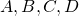describing the  modes of the hybrid  system are generated randomly by using the rss() Matlab function.  The  generated systems are then stabilized with an LQR controller to garantee a convergence to a stable attracting region. The transitions are determined heuristically by means of simulations.
The proposed benchmark consists of 5 modes und 5 transitions. The continuous dynamics in each mode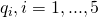is described by the following ODE: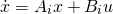where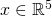is the state vector,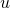is an input signal confined  in  compact bounded set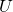and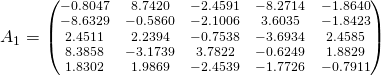,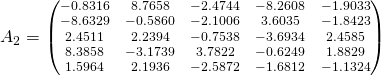,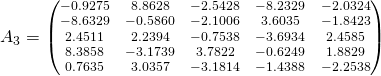,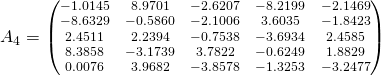,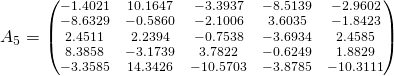,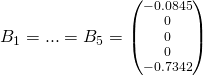,

The corresponding hybrid automaton is given in fig.1.

## Reachability settings

We use the initial set

•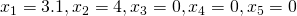•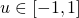with the initial location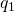. The time horizion is set to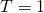.

## Results

SpaceEx results for a time horizon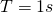and box directions :